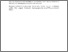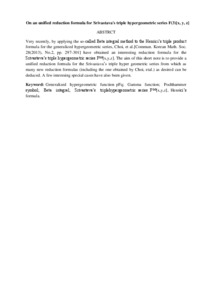# On an unified reduction formula for Srivastava's triple hypergeometric series F(3)[x, y, z]

## Citation

Yong, Sup Kim and Kilicman, Adem and Rathie, A. K. (2018) On an unified reduction formula for Srivastava's triple hypergeometric series F(3)[x, y, z]. New Trends in Mathematical Sciences, 6 (2). pp. 297-301. ISSN 2147-5520

## Abstract

Very recently, by applying the so-called Beta integral method to the Henrici’s triple product formula for the generalized hypergeometric series, Choi, et al.[Commun. Korean Math. Soc. 28(2013), No.2, pp. 297-301] have obtained an interesting reduction formula for the Srivastava’s triple hypergeometric series F⁽ᶟ⁾[x,y,z]. The aim of this short note is to provide a unified reduction formula for the Srivastava’s triple hyper geometric series from which as many new reduction formulas (including the one obtained by Choi, etal.) as desired can be deduced. A few interesing special cases have also been given.Preview
Text
On an unified.pdfView Item